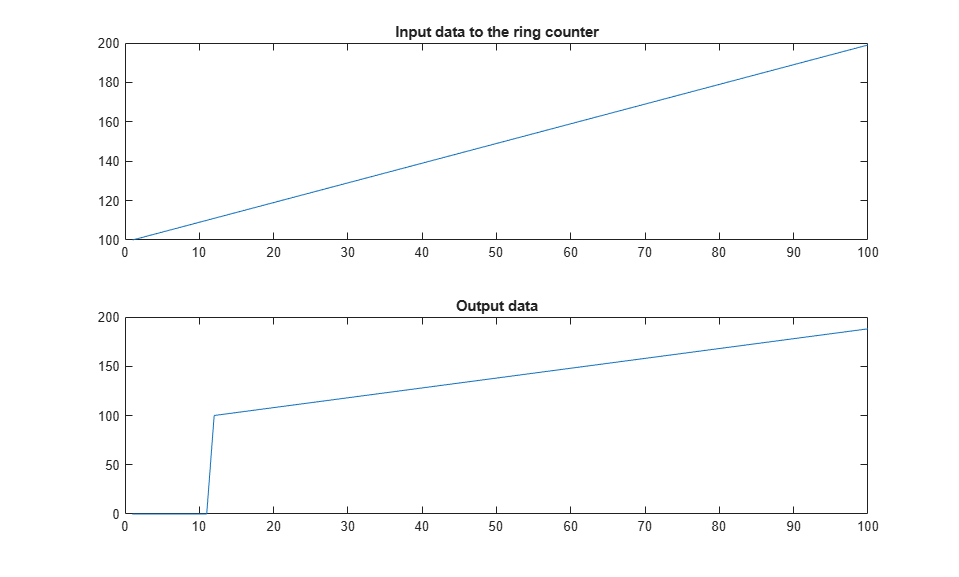# HDL Code Generation from hdl.RAM System Object

This example shows how to generate HDL code from MATLAB® code from hdl.RAM System object™ in MATLAB and infer RAM in generated hardware.

### MATLAB Design

This example shows implementation of a line delay that uses a memory in a ring structure, where data is written in one position and read from another position in such a way that the data written will be read after a specific number of cycles. An efficient implementation of this architecture on Virtex FPGAs uses the on-chip Dual Port Block RAMs and an address counter. The Block RAMs can be configured as 512x8 or 256x9 synchronous Dual Port RAMs. To parameterize the delay length, the RAM write address is generated by a counter and the read address is generated by adding a constant K to the write address. If the memory size is M, the input will be read M-K clock cycles after it was written to the memory, hence implementing M-K word shift behaviour.

```design_name = 'mlhdlc_hdlram'; testbench_name = 'mlhdlc_hdlram_tb'; ```

Look at the MATLAB design.

```type(design_name); ```
```%#codegen function data_out = mlhdlc_hdlram(data_in) % % This example shows implementation of a line delay that uses a memory in a % ring structure, where data is written in one position and read from % another position in such a way that the data written will be read after a % specific number of cycles. An efficient implementation of this % architecture on Virtex FPGAs uses the on-chip Dual Port Block RAMs and an % address counter. The Block RAMs can be configured as 512x8 or 256x9 % synchronous Dual Port RAMs. To parameterize the delay length, the RAM % write address is generated by a counter and the read address is generated % by adding a constant K to the write address. If the memory size is M, the % input will be read M-K clock cycles after it was written to the memory, % hence implementing M-K word shift behaviour. % Copyright 2012-2015 The MathWorks, Inc. persistent hRam; if isempty(hRam) hRam = hdl.RAM('RAMType', 'Dual port'); end % read address counter persistent rdAddrCtr; if isempty(rdAddrCtr) rdAddrCtr = 0; end % ring counter length ringCtrLength = 10; ramWriteAddr = rdAddrCtr + ringCtrLength; ramWriteData = data_in; ramWriteEnable = true; ramReadAddr = rdAddrCtr; % execute single step of RAM [~, ramRdDout] = step(hRam, ramWriteData, ramWriteAddr, ramWriteEnable, ramReadAddr); rdAddrCtr = rdAddrCtr + 1; data_out = ramRdDout; ```
```type(testbench_name); ```
```function mlhdlc_hdlram_tb % % Copyright 2012-2015 The MathWorks, Inc. clear test_hdlram; data = 100:200; ring_out = zeros(1, length(data)); for ii=1:100 ring_in = data(ii); ring_out(ii) = mlhdlc_hdlram(ring_in); end figure('Name', [mfilename, '_plot']); subplot(2,1,1); plot(1:100,data(1:100)); title('Input data to the ring counter') subplot(2,1,2); plot(1:100,ring_out(1:100)); title('Output data') end ```

### Create a New Folder and Copy Relevant Files

Execute the following lines of code to copy the necessary example files into a temporary folder.

```mlhdlc_demo_dir = fullfile(matlabroot, 'toolbox', 'hdlcoder', 'hdlcoderdemos', 'matlabhdlcoderdemos'); mlhdlc_temp_dir = [tempdir 'mlhdlc_sysobj_intro']; % Create a temporary folder and copy the MATLAB files. cd(tempdir); [~, ~, ~] = rmdir(mlhdlc_temp_dir, 's'); mkdir(mlhdlc_temp_dir); cd(mlhdlc_temp_dir); copyfile(fullfile(mlhdlc_demo_dir, [design_name,'.m*']), mlhdlc_temp_dir); copyfile(fullfile(mlhdlc_demo_dir, [testbench_name,'.m*']), mlhdlc_temp_dir); ```

### Simulate the Design

Simulate the design with the test bench prior to code generation to make sure there are no runtime errors.

```mlhdlc_hdlram_tb ```### Create a New HDL Coder™ Project

To create a new project, enter the following command:

```coder -hdlcoder -new mlhdlc_sysobj_prj ```

Next, add the file `mlhdlc_hdlram.m` to the project as the MATLAB Function and `mlhdlc_hdlram_tb.m` as the MATLAB Test Bench.

For a more complete tutorial on creating and populating MATLAB HDL Coder projects, see Get Started with MATLAB to HDL Workflow.

### Run Fixed-Point Conversion and HDL Code Generation

Launch the Workflow Advisor. In the Workflow Advisor, right-click the 'Code Generation' step. Choose the option 'Run to selected task' to run all the steps from the beginning through HDL code generation.

Examine the generated HDL code by clicking the links in the log window.

### Supported System objects

For a list of System objects supported for HDL code generation, see Predefined System Objects Supported for HDL Code Generation.

### Clean up the Generated Files

Run the following commands to clean up the temporary project folder.

```mlhdlc_demo_dir = fullfile(matlabroot, 'toolbox', 'hdlcoder', 'hdlcoderdemos', 'matlabhdlcoderdemos'); mlhdlc_temp_dir = [tempdir 'mlhdlc_sysobj_intro']; clear mex; cd (mlhdlc_demo_dir); rmdir(mlhdlc_temp_dir, 's'); ```